# Seeing Stars

Let us now take a closer look at the hex-fractal we sliced last week. Chopping a level 0, 1, 2, and 3 Menger sponge through our slanted plane gives the following:

This suggests an iterative recipe to generate the hex-fractal. Any time we see a hexagon, chop it into six smaller hexagons and six triangles as illustrated below. Similarly, any time we see a triangle, chop it into a hexagon and three triangles like this:A recipe for constructing the hex-fractal: replace each hexagon with 6 hexagons and 6 triangles, and replace each triangle with 1 hexagon and 3 triangles.

In the limit, each triangle and hexagon in the above image becomes a hex-fractal or a tri-fractal, respectively. The final hex-fractal looks something like this (click for larger image):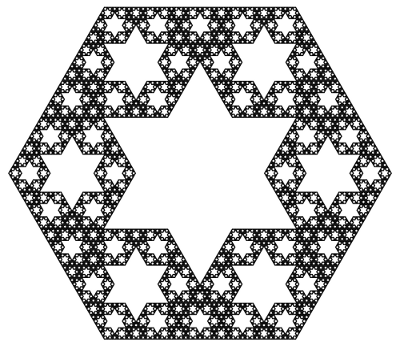Now we are in a position to answer last week’s question: how can we compute the Hausdorff dimension of the hex-fractal? Let d be its dimension. Like last week, our computation will proceed by trying to compute the “d-dimensional volume” of our shape. So, start with a “large” hex-fractal and tri-fractal, each of side-length 1, and let their d-dimensional volumes be h and t respectively.

Break these into “small” hex-fractals and tri-fractals of side-length 1/3, so these have volumes $$h/3^d$$ and $$t/3^d$$ respectively (this is how “d-dimenional stuff” scales). Since $$\begin{gather*}(\text{large hex}) = 6(\text{small hex})+6(\text{small tri}) \quad \text{and}\\ (\text{large tri}) = (\text{small hex})+3(\text{small tri}),\end{gather*}$$ we find that $$h=6h/3^d + 6t/3^d$$ and $$t=h/3^d+3t/3^d$$. Surprisingly, this is enough information to solve for the value of $$3^d$$. We find $$3^d = \frac{1}{2}(9+\sqrt{33})$$, so $$d=\log_3\left(\frac{9+\sqrt{33}}{2}\right) = 1.8184\ldots,$$ as claimed last week.

As a final thought, why did we choose to slice the Menger sponge on this plane? Why not any of the (infinitely many) others? Even if we only look at planes parallel to our chosen plane, a mesmerizing pattern emerges:

It takes a bit more work to turn the above computation of the hex-fractal’s dimension into a full proof, but there are a few ways to do it. Possible methods include mass distributions or similarity graphs.

This diagonal slice through the Menger sponge has been proposed as an exhibit at the Museum of Math. Sebastien Perez Duarte seems to have been the first to slice a Menger sponge in this way (see his rendering), and his animated cross section inspired my animation above.

### Notes

1. We’re assuming that the hex-fractal and tri-fractal have the same Hausdorff dimension. This is true, and it follows from the fact that a scaled version of each lives inside the other. []
2. There are actually two solutions, but the fact that h and t are both positive rules one out. []
3. Proposition 4.9 in: Kenneth Falconer. Fractal Geometry: Mathematical Foundations and Applications. John Wiley & Sons: New York, 1990. []
4. Section 6.6 in: Gerald Edgar. Measure, Topology, and Fractal Geometry (Second Edition). Springer: 2008. []

# A Slice of Interdimensional Sponge Cake

The Menger sponge is a delightfully pathological shape to soak up. Start with a cube of side-length 1, and “hollow it out” by slicing it into 27 sub-cubes (like a Rubik’s Cube) and removing the 7 central sub-cubes as illustrated below. Call this the level 1 sponge. To make the 2nd level, perform the same “hollowing out” operation on each of the 20 cubes in level 1. Keep going to make levels 3, 4, and so on. The end result, i.e., level $$\infty$$, is a fractal known as the Menger Sponge. It has a crazy network of tunnels and passageways running through it: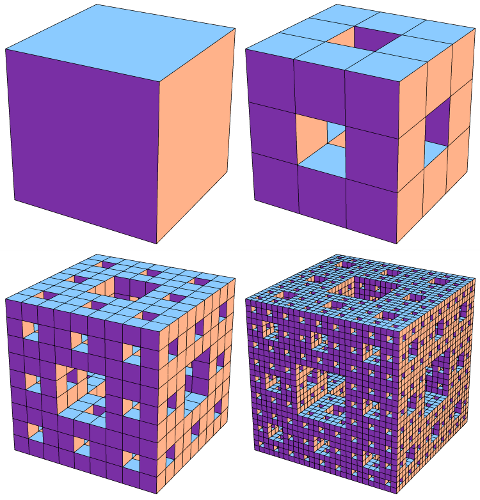Stages in the construction of the Menger sponge: Levels 0, 1, 2, and 3. Click for larger image.

What is its volume? We made the Menger Sponge by cutting out smaller and smaller chunks from the original unit cube, so how much volume did we leave behind? Each “hollowing out” step reduces the volume by a factor of 20/27, so level k has volume $$(20/27)^k$$ and the final Menger Sponge has volume 0. We removed all the volume!

Since the Menger Sponge doesn’t have enough “3D stuff” to be considered a truly 3-dimensional shape, maybe it behaves more like a 2-dimensional surface? This isn’t quite right either. The Menger Sponge has “too much 2D stuff”: its surface area is infinite. In fact, this shape falls squarely (hah!) in the middle: it has dimension 2.7268….

Wait, what does it mean to have non-integer dimension? One interpretation, formalized in the notion of Hausdorff dimension, is to look at how the “stuff” it is made from behaves under scaling. If we take a square (which is made of “2-dimensional stuff”) and scale it by a factor of 1/3, its area changes by a factor of $$(1/3)^2$$. Similarly, scaling a cube (“3D stuff”) by 1/3 scales its volume by $$(1/3)^3$$. In general, scaling “d-dimensional stuff” by a factor of 1/3 scales its “d-dimensional volume” by $$(1/3)^d$$.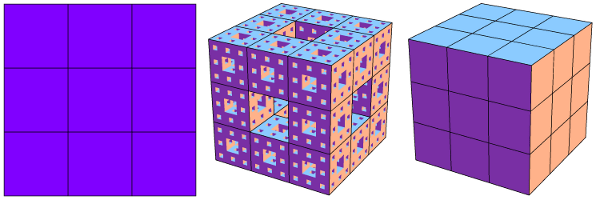How different dimensional "stuff" behaves when scaled by a factor of 1/3. Left: A 2D plane is split into 9=3^2 smaller copies. Right: A 3D cube is split into 27=3^3 copies. Middle: A Menger sponge is split into 20 smaller copies.

As shown in this image, a Menger Sponge can be chopped into 20 smaller Menger Sponges, each scaled by 1/3. So if the Menger Sponge is made of m-dimensional stuff, its m-dimensional volume scales by 1/20 when the shape is scaled by 1/3, so we should have $$(1/3)^m=1/20$$, i.e., $$m=\log_3(20) = 2.7268\ldots$$.

As one more example of the quirkiness that can be squeezed out of the Menger Sponge, what happens when we slice this shape along one of its primary diagonal planes? The Menger Sponge’s crazy network of tunnels creates a stellar array of 6-pointed stars cut out of a regular hexagon: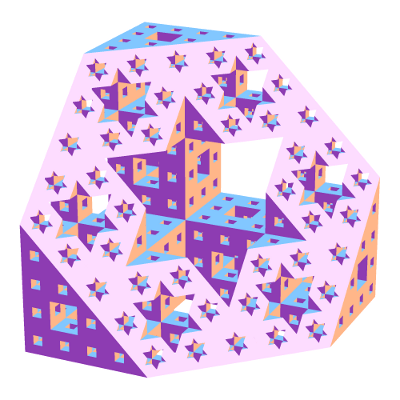Slicing a Menger sponge along a primary diagonal plane produces a dazzling fractal (living in the slicing plane) made from 6-pointed stars.

What is the Hausdorff dimension of this hexagonal fractal? It turns out to be $$\log_3\left(\tfrac{9+\sqrt{33}}{2}\right) = 1.8184\ldots.$$ To find out where this ridiculous number comes from, you’ll have to come back next week!

### Notes

1. This is a technical term, of course. []
2. The surface area of level k can be computed to be $$2\cdot(20/9)^k+4\cdot(8/9)^k$$, and this approaches infinity as k grows. []
3. Specifically, slice it along the perpendicular-bisecting plane of one of the cube’s diagonals []

# Spherical Surfaces and Hat Boxes

To round off our series on round objects (see the first and second posts), let’s compute the sphere’s surface area. We can compute this in the same way we related the area and circumference of a circle two weeks ago. Approximate the surface of the sphere with lots of small triangles, and connect these to the center of the sphere to create lots of triangular pyramids. Each pyramid has volume $$\frac{1}{3}(\text{area of base})(\text{height})$$, where the heights are all nearly $$r$$ and the base areas add to approximately the surface area. By using more and smaller triangles these approximations get better and better, so the volume of the sphere is $$\frac{4}{3}\pi r^3 = \frac{1}{3}(\text{surface area})\cdot r,$$ meaning the surface area is $$4\pi r^2$$. (This and previous arguments can be made precise with the modern language of integral calculus.)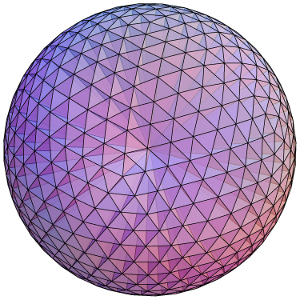Dividing a sphere into many pyramids connected to the center allows us to relate the sphere's surface area and volume.

Here’s an elegant way to rephrase this result: The surface area of a sphere is equal to the area of the curved portion of a cylinder that exactly encloses the sphere. In fact, something very surprising happens here!:

Archimedes’ Hat-Box Theorem: If we draw any two horizontal planes as shown below, then the portions of the sphere and the cylinder between the two planes have the same surface area.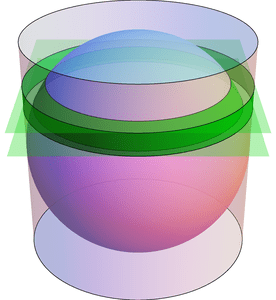Any two horizontal planes cut off a band on the sphere and another band on the enclosing cylinder. Archimedes' Hat-Box Theorem says that these bands have the same area. (The planes shown here have heights $$0.4\cdot r$$ and $$0.6\cdot r$$ above the equator.)

We can prove this with (all!) the methods in the last few posts; here’s a quick sketch. To compute the area of the “spherical band” (usually called a spherical zone), first consider the solid spherical sector formed by joining the spherical zone to the center: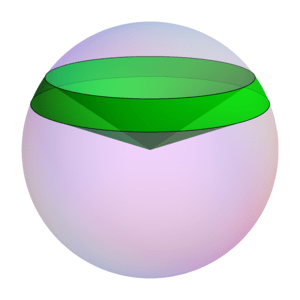The Hat-Box theorem can be proved by relating the area of the spherical zone to the volume of this spherical sector.

By dividing this into lots of triangular pyramids as we did with the sphere above, we can compute the area of the spherical zone by instead computing the sector’s volume. This volume can be computed by breaking it into three parts: two cones and the spherical segment between the two planes (on the left of the next figure). Compute the volume of the spherical segment by comparing (via Cavalieri’s Principle) to the corresponding part of the vase (from the previous post), which can be expressed with just cylinders and cones.

See if you can fill in the details!

# Slicing Spheres

Last week we saw how to compute the area of a circle from first principles. What about spheres?

To compute the volume of a sphere, let’s show that a hemisphere (with radius $$r$$) has the same volume as the vase shown in the figure below, formed by carving a cone from the circular cylinder with radius and height $$r$$. Why this shape? Here’s why: if we cut these two solids at any height $$h$$ (between 0 and $$r$$), the areas of the two slices match. Indeed, the slice—usually called cross section—of the sphere is a circle of radius $$\sqrt{r^2-h^2}$$, which has area $$\pi(r^2-h^2)$$. Similarly, the vase’s cross section is a radius $$r$$ circle with a radius $$h$$ circle cut out, so its area is $$\pi r^2-\pi h^2$$, as claimed.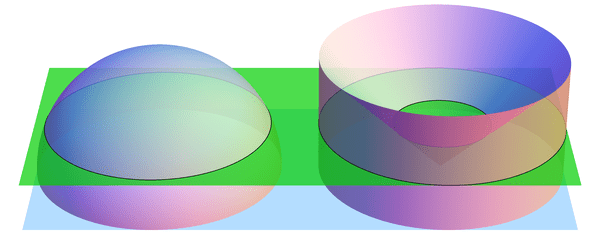When sliced by a horizontal plane at any height $$h$$, the hemisphere and vase have equal cross-sectional areas. (Shown here for $$h = 0.4\cdot r$$.) By Cavalieri's Principle, this implies that they have equal volumes.

If we imagine the hemisphere and vase as being made from lots of tiny grains of sand, then we just showed, intuitively, that the two solids have the same number of grains of sand in every layer. So there should be the same number of grains in total, i.e., the volumes should match. This intuition is exactly right:

Cavalieri’s Principle: any two shapes that have matching horizontal cross sectional areas also have the same volume.

So the volumes are indeed equal, and all that’s left is to compute the volume of the vase. But we can do this! Recall that the cone has volume $$\frac{1}{3} (\text{area of base}) (\text{height}) = \frac{1}{3}\pi r^3$$ (better yet, prove this too! Hint: use Cavalieri’s Principle again to compare to a triangular pyramid). Likewise, the cylinder has volume $$(\text{area of base}) (\text{height}) = \pi r^3$$, so the vase (and hemisphere) have volume $$\pi r^3 – \frac{1}{3} \pi r^3 = \frac{2}{3}\pi r^3$$. The volume of the whole sphere is thus $$\frac{4}{3}\pi r^3$$. Success!

The following visualization illustrates what we have shown, namely $$\text{hemisphere} + \text{cone} = \text{cylinder}.$$ The “grains of sand” in the hemisphere are being displaced horizontally by the stabbing cone, and at the end we have exactly filled the cylinder.

# Archimedes’ Circular Reasoning

Every geometry textbook has formulas for the circumference ($$C = 2 \pi r$$) and area ($$A = \pi r^2$$) of a circle. But where do these come from? How can we prove them?

Well, the first is more a definition than a theorem: the number $$\pi$$ is usually defined as the ratio of a circle’s circumference to its diameter: $$\pi = C/(2r)$$. Armed with this, we can compute the area of a circle. Archimedes’ idea (in 260 BCE) was to approximate this area by looking at regular $$n$$-sided polygons drawn inside and outside the circle, as in the diagram below. Increasing $$n$$ gives better and better approximations to the area.The n-sided regular polygons inside (blue) and outside (red) a circle can be used to approximate the area of the circle. Shown here for n=12.

Look first at the inner polygon. Its perimeter is slightly less than the circle’s circumference, $$C = 2 \pi r$$, and the height of each triangle is slightly less than $$r$$. So when reassembled as shown, the triangles form a rectangle whose area is just under $$C/2\cdot r = \pi r^2$$. Likewise, the outer polygon has area just larger than $$\pi r^2$$. As $$n$$ gets larger, these two bounds get closer and closer to $$\pi r^2$$, which is therefore the circle’s area.

Archimedes used this same idea to approximate the number $$\pi$$. Not only was he working by hand, but the notion of “square root” was not yet understood well enough to compute with. Nevertheless, he was amazingly able to use 96-sided polygons to approximate the circle! His computation included impressive dexterity with fractions: for example, instead of being able to use $$\sqrt{3}$$ directly, he had to use the (very close!) approximation $$\sqrt{3} > 265/153$$. In the end, he obtained the bounds $$3\frac{10}{71} < \pi < 3\frac{1}{7}$$, which are accurate to within 0.0013, or about .04%. (In fact, he proved the slightly stronger but uglier bounds $$3\frac{1137}{8069} < \pi < 3\frac{1335}{9347}$$. See this translation and exposition for more information on Archimedes’ methods.)

These ideas can be pushed further. Focus on a circle with radius 1. The area of the regular $$n$$-sided polygon inscribed in this circle can be used as an approximation for the circle’s area, namely $$\pi$$. This polygon has area $$A_n = n/2 \cdot \sin(360/n)$$ (prove this!). What happens when we double the number of sides? The approximation changes by a factor of $$\frac{A_{2n}}{A_n} = \frac{2\sin(180/n)}{\sin(360/n)} = \frac{1}{\cos(180/n)}.$$ Starting from $$A_4 = 2$$, we can use the above formula to compute $$A_8,A_{16},A_{32},\ldots$$, and in the limit we find that $$\pi = \frac{2}{\cos(180/4)\cdot\cos(180/8)\cdot\cos(180/16)\cdots}.$$ Finally, recalling that $$\cos(180/4) = \cos(45) = \sqrt{\frac{1}{2}}$$ and $$\cos(\theta/2) = \sqrt{\frac{1}{2}(1+\cos\theta)}$$ (whenever $$\cos(\theta/2) \ge 0$$), we can rearrange this into the fun infinite product $$\frac{2}{\pi} = \sqrt{\frac{1}{2}} \cdot \sqrt{\frac{1}{2}+\frac{1}{2}\sqrt{\frac{1}{2}}} \cdot \sqrt{\frac{1}{2}+\frac{1}{2}\sqrt{\frac{1}{2}+\frac{1}{2}\sqrt{\frac{1}{2}}}} \cdots$$ (which I found at Mathworld). (It’s ironic that this formula for a circle uses so many square roots!)

# Some Strange Sums

If someone told you to compute the value of the infinite sum $$Z = 1+2+3+4+\cdots$$, you would tell them that it simply does not converge. But what if they insisted that you assign a finite value to this sum? Which value would you give? Is there any way to make sense of this question? Yes, and the answer is $$-1/12$$.

Before tackling this sum, let’s try a slightly easier one, known as Grandi’s Series: $$G = 1-1+1-1+\cdots$$. How can we compute its value? Let’s write \begin{align*}G &= 1-1+1-1+1-\cdots \text{ and}\\ G &= 0+1-1+1-1+\ldots,\end{align*} and adding these term by term gives $$2G = 1+0+0+0+0+\cdots = 1$$, i.e., $$G = 1/2$$. So we got a value! The same “trick” lets us sum $$H = 1-2+3-4+\cdots$$ like this: \begin{align*}2H &= 1 + (-2+1) + (3-2) + (-4+3) + \cdots\\ &= 1-1+1-1+\cdots\\ &= G = 1/2,\end{align*} so $$H = 1/4$$.

What’s really going on here? A good way to understand it is to look at the functions $$g(x) = 1-x+x^2-x^3+\cdots$$ and $$h(x) = 1-2x+3x^2-4x^3+\cdots$$, and to realize that we’re asking for $$g(1)$$ and $$h(1)$$, respectively. The sum defining $$g(x)$$ converges for $$-1 < x < 1[/latex], and since $g(x) + x \cdot g(x) = 1$ (this is just the "trick" from above!), we find $g(x) = 1/(1+x)$. So, even though the sum defining $g(1) = 1-1+1-\cdots$ does not converge, the limit as $x$ approaches 1 from below is well defined and equals $1/(1+1) = 1/2$. Likewise, we find that $h(x) = 1/(1+x)^2$ for $-1 < x < 1$, and the limit as $x$ goes to 1 is 1/4. This method of putting the terms as the coefficients of a power series and extrapolating with limits is called Abel Regularization. Notice, however, that this method does not work for the sum [latex]Z$$, because $$1 + 2x + 3x^2 + 4x^3 + \cdots = 1/(1-x)^2$$, and the limit as $$x$$ approaches 1 is still infinite.

A different method of summing divergent series is called Zeta Function Regularization: to compute $$a_1 + a_2 + a_3 + \cdots$$, define the function $$a_1^{-s} + a_2^{-s} + a_3^{-s} + \cdots$$ (which hopefully converges for large enough s), and try to “extend” this to a function we can evaluate at $$s=-1$$. For example, for $$H = 1-2+3-\cdots$$, the corresponding function $$1/1^2-1/2^s+1/3^s-\cdots$$ is called the Dirichlet Eta Function, $$\eta(s)$$. Analysis based on our “trick” from above (iterated infinitely many times! [producing the so-called Euler Transform]) can be used to show that $$\eta(s)$$ can be defined for all values $$s$$ (even complex values!) and that $$\eta(-1) = 1/4$$, which agrees with our computation of $$1-2+3-4+\cdots$$ above. The corresponding function for the sum $$Z$$ is called the Riemann Zeta Function, $$\zeta(s)$$, and we can compute (for all $$s$$ where this makes sense): $$\begin{gather*} \zeta(s) = \frac{1}{1^s} + \frac{1}{2^s} + \frac{1}{3^s} + \frac{1}{4^s} + \cdots,\\ 2^{-s} \zeta(s) = \frac{1}{2^s} + \frac{1}{4^s} + \frac{1}{6^s} + \frac{1}{8^s} + \cdots, \text{ and so}\\ \zeta(s)-2\cdot 2^{-s} \zeta(s) = \frac{1}{1^s}-\frac{1}{2^s}+\frac{1}{3^s}-\frac{1}{4^s}+\cdots = \eta(s).\end{gather*}$$ So $$(1-2^{1-s}) \zeta(s)$$ equals $$\eta(s)$$ everywhere (by general complex analysis facts). At $$s = -1$$, this says $$\zeta(-1) = \eta(-1)/(-3) = -1/12$$, as claimed.